# Solution Sheets

### Hypothesis Testing with One Sample

Class Time: __________________________
Name: _____________________________________

1. H0: _______
2. Ha: _______
3. In words, CLEARLY state what your random variable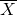or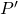represents.
4. State the distribution to use for the test.
5. What is the test statistic?
6. What is the p-value? In one or two complete sentences, explain what the p-value means for this problem.
7. Use the previous information to sketch a picture of this situation. CLEARLY, label and scale the horizontal axis and shade the region(s) corresponding to the p-value.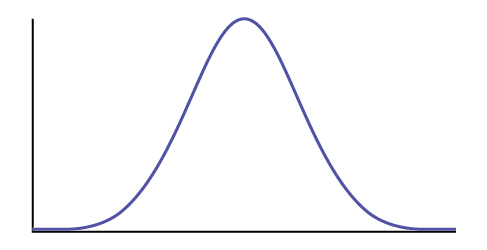8. Indicate the correct decision (“reject” or “do not reject” the null hypothesis), the reason for it, and write an appropriate conclusion, using complete sentences.
1. Alpha: _______
2. Decision: _______
3. Reason for decision: _______
4. Conclusion: _______
9. Construct a 95% confidence interval for the true mean or proportion. Include a sketch of the graph of the situation. Label the point estimate and the lower and upper bounds of the confidence interval.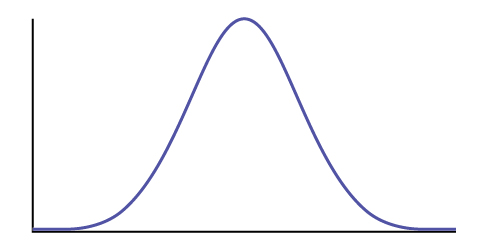### Hypothesis Testing with Two Samples

Class Time: __________________________
Name: _____________________________________

1. H0: _______
2. Ha: _______
3. In words, clearly state what your random variable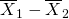,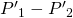or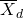represents.
4. State the distribution to use for the test.
5. What is the test statistic?
6. What is the p-value? In one to two complete sentences, explain what the p-value means for this problem.
7. Use the previous information to sketch a picture of this situation. CLEARLY label and scale the horizontal axis and shade the region(s) corresponding to the p-value.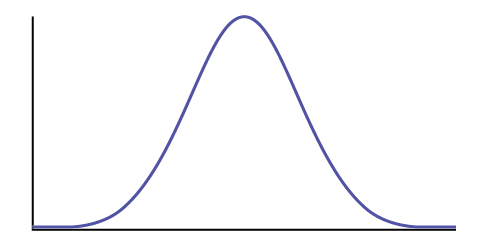8. Indicate the correct decision (“reject” or “do not reject” the null hypothesis), the reason for it, and write an appropriate conclusion, using complete sentences.
1. Alpha: _______
2. Decision: _______
3. Reason for decision: _______
4. Conclusion: _______
9. In complete sentences, explain how you determined which distribution to use.

### The Chi-Square Distribution

Class Time: __________________________
Name: ____________________________________

1. H0: _______
2. Ha: _______
3. What are the degrees of freedom?
4. State the distribution to use for the test.
5. What is the test statistic?
6. What is the p-value? In one to two complete sentences, explain what the p-value means for this problem.
7. Use the previous information to sketch a picture of this situation. Clearly label and scale the horizontal axis and shade the region(s) corresponding to the p-value.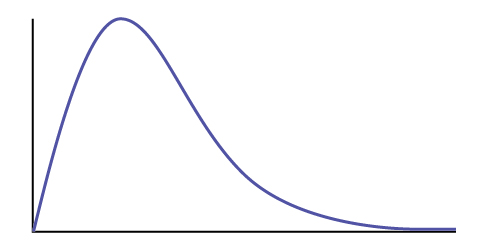8. Indicate the correct decision (“reject” or “do not reject” the null hypothesis) and write appropriate conclusions, using complete sentences.
1. Alpha: _______
2. Decision: _______
3. Reason for decision: _______
4. Conclusion: _______

### F Distribution and One-Way ANOVA

Class Time: __________________________
Name: ____________________________________

1. H0: _______
2. Ha: _______
3. df(n) = ______ df(d) = _______
4. State the distribution to use for the test.
5. What is the test statistic?
6. What is the p-value?
7. Use the previous information to sketch a picture of this situation. Clearly label and scale the horizontal axis and shade the region(s) corresponding to the p-value.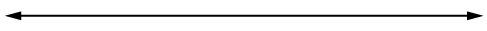8. Indicate the correct decision (“reject” or “do not reject” the null hypothesis) and write appropriate conclusions, using complete sentences.
1. Alpha: _______
2. Decision: _______
3. Reason for decision: _______
4. Conclusion: _______